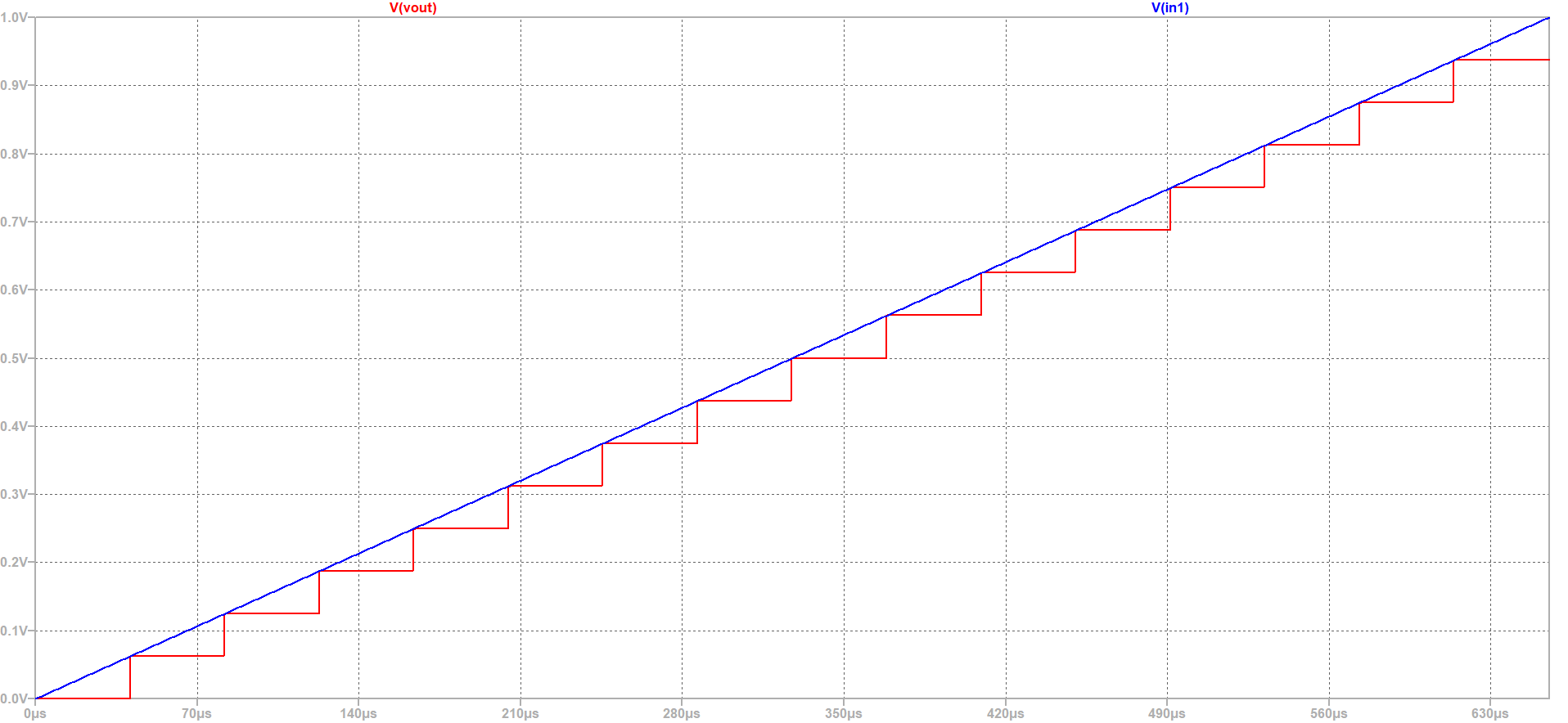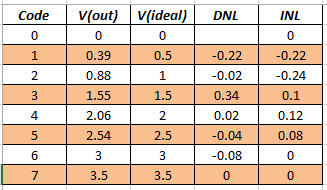# Overview

• Motivation
• Copy of zip files for web report
• LTSPICE
• RC circuit drawing
• Simulations: .OP; .tran; .DC; .AC
• Create a web report
• Simulate a behavioral ADC, DAC with LTSPICE
• Summary
• References

# Motivation

The purpose of this lab is to familiarize with web lab report.
In this lab we have covered Analog to Digital Converter (ADC) and Digital to Analog Converter (DAC).
We have done our simulation by using LTSpice Software.
We have also calculated DNL (Differential Nonlinearity) and INL (Integral Nonlinearity) of our desired transfer curve.

# Install Webpage Template

• Download the Template .zip file
• Copy of zip files for web report
• Unzip the file
• Copy Directory 2019_GroupX to 2020_GroupC2_Likhon
• In the Directory Copy the file: 2017_Group01_V00.html to 2020_GroupC2_Likhon
• Edit: With Notepad:2020_GroupC2_Likhon
• Change the Header, Change Footer, Made Slide View, Installation

# Test for 4 Bit ADC and DAC

 A 4 Bit ADC and DAC test can be simulated in LTSPICE. The files were downloaded and LTSpice simulation was started. The output file size can be limited by using the .save dialog option. A voltage source was added with a ramp from 0 to 1V with a rise time of 655µs. The picture showa ramp input voltages and the DAC ramp output voltage over time 16 steps can be seen with a measurement statement the voltage level were extracted. .Measure TRAN V0000 FIND V(Vout)AT=20u At 60us time the output of 0.0625 V is given for the code 0001. V0001:V0001: V(Vout)=0.0625 at 6e-005 No error in the voltage level can be seen. It is an ideal ADC and DAC. Measurement Codes: .Measure TRAN V0001 FIND V(Vout)AT=60u .Measure TRAN V0002 FIND V(Vout)AT=100u .Measure TRAN V0003 FIND V(Vout)AT=140u .Measure TRAN V0004 FIND V(Vout)AT=180u .Measure TRAN V0005 FIND V(Vout)AT=220u .Measure TRAN V0006 FIND V(Vout)AT=260u .Measure TRAN V0007 FIND V(Vout)AT=300u .Measure TRAN V0008 FIND V(Vout)AT=340u .Measure TRAN V0009 FIND V(Vout)AT=380u .Measure TRAN V0010 FIND V(Vout)AT=420u .Measure TRAN V0011 FIND V(Vout)AT=460u .Measure TRAN V0012 FIND V(Vout)AT=500u .Measure TRAN V0013 FIND V(Vout)AT=540u .Measure TRAN V0014 FIND V(Vout)AT=580u .Measure TRAN V0015 FIND V(Vout)AT=620u# LTSPICE schematicsStep size: LSB = V(111)−V(000)/(2^3)−1 = (3.5-0)/7 = 0.5 The step size between 2 successive codes is normalized with LSB and calculated as DNL: DNL(n) = V(n)−V(n−1)−LSB/LSB

# Summery

While writing the web lab report I faced some problems.
1. LTSpice Circuit diagram and simulation graph were not appearing on the web page by using chrome browser.
Solved: After installing Firefox this problem solved.
2. My LTSpice Schematic WS2011_Prob4.asc was not appearing on the web page.
Solved: Switch.asc and Switch.asy files were in different folders. After putting them on the same folder the schematic appeared on the web page.